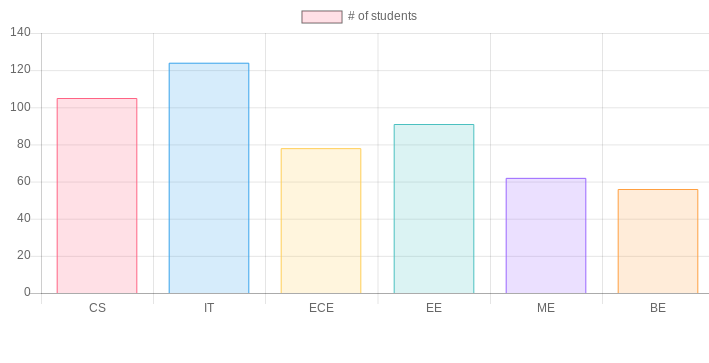# JavaScript | Chart.js

Chart.js is an open source JavaScript library on Github that allows you to draw different types of charts by using the HTML5 canvas element. Since it uses canvas, you have to include a polyfill to support older browsers.

So, what is HTML5 Canvas Element ?

The HTML5 element gives an easy and powerful way to draw graphics using JavaScript. It can be used to draw graphs, make photo compositions or do simple (and not so simple) animations.
It is responsive in nature that means it redraws chart on resizing of window for perfect scale granularity.

This library supports 8 different types of graphs :

1. Line
2. Bar
3. Doughnut
4. Pie
6. Polar Area
7. Bubble
8. Scatter

Steps to create a chart:

1. First include the chart.js in the HTML.

 `<``head``> ` `<``script` `src``= ` `"https://cdnjs.cloudflare.com/ajax/libs/Chart.js/2.7.2/Chart.bundle.js"``> ` ` ` `<``link` `rel``=``"stylesheet"` `type``=``"text/css"` `href``=``"style.css"``> ` ` `

2. Create canvas: To create a chart we need to represent the Chart class. In order to do this, we need to pass jQuery instance or 2d context of the canvas of where we want the place or draw the chart.

For example:

 `<``canvas` `id = ”chart” width=”900” height = “900”> `

3. Type of Chart and Data: Decide what type of chart is required and prepare the data accordingly. Data requires labels, datasets, data values, backgroundColor,borderColor, borderWidth and various other options.

For example:

 `labels:[“CS”, “IT” , “ECE” , “EE”, ”ME”, “BE”], ` `And datasets:  ` `    ``Label: ‘``# of students’, ` `    ``Data : [105,124,78,91,62,56], ` `    ``backgroundColor :[``'rgba(255, 99, 132, 0.2)'``, ` `                ``'rgba(54, 162, 235, 0.2)'``, ` `                ``'rgba(255, 206, 86, 0.2)'``, ` `                ``'rgba(75, 192, 192, 0.2)'``, ` `                ``'rgba(153, 102, 255, 0.2)'``, ` `                ``'rgba(255, 159, 64, 0.2)'` `], ` ` `  `borderColor: [ ` `                ``'rgba(255,99,132,1)'``, ` `                ``'rgba(54, 162, 235, 1)'``, ` `                ``'rgba(255, 206, 86, 1)'``, ` `                ``'rgba(75, 192, 192, 1)'``, ` `                ``'rgba(153, 102, 255, 1)'``, ` `                ``'rgba(255, 159, 64, 1)'` `            ``] `

And finally, we should decide the type of chart from a line, bar, radar, pie, doughnut, polar area, bubble and scatter.

4. Create a graph: After defining what type of graph is to be drawn, pass the data to that graph that we want to visualize. Below is an example:

 `var` `ctx = document.getElementById(``"chart"``); ` `var` `myChart = ``new` `Chart(ctx, { ` `  ``type: ``'bar'``, ` `  ``data: { ` `    ``Labels: [“CS”, “IT” , “ECE” , “EE”, ”ME”, “BE”], ` `    ``datasets: [ ` `      ``{ ` `       ``label: ‘``# of students’, ` `    ``data : [105,124,78,91,62,56], ` `    ``backgroundColor :[``'rgba(255, 99, 132, 0.2)'``, ` `                ``'rgba(54, 162, 235, 0.2)'``, ` `                ``'rgba(255, 206, 86, 0.2)'``, ` `                ``'rgba(75, 192, 192, 0.2)'``, ` `                ``'rgba(153, 102, 255, 0.2)'``, ` `                ``'rgba(255, 159, 64, 0.2)'` `], ` ` `  `borderColor: [ ` `                ``'rgba(255,99,132,1)'``, ` `                ``'rgba(54, 162, 235, 1)'``, ` `                ``'rgba(255, 206, 86, 1)'``, ` `                ``'rgba(75, 192, 192, 1)'``, ` `                ``'rgba(153, 102, 255, 1)'``, ` `                ``'rgba(255, 159, 64, 1)'` `            ``], ` `borderWidth : 1 ` ` `  `} ` `      ``} ` `    ``] `

Complete code to create a chart:

 ` ` `<``html``> ` `<``head``> ` `<``script`  `src``=``"https://cdnjs.cloudflare.com/ajax/libs/Chart.js/2.7.2/Chart.bundle.js"``> ` ` ` ` ` `<``body``> ` ` `  `<``canvas` `id``=``"myChart"` `width``=``"900"` `height``=``"400"``> ` `<``script` `type``=``"text/javascript"``> ` ` `  `var ctx = document.getElementById("myChart"); ` `var myChart = new Chart(ctx, { ` `  ``type: 'bar', ` `  ``data: { ` `    ``labels: ["CS", "IT" , "ECE" , "EE", "ME", "BE"], ` `    ``datasets: [ ` `      ``{ label: '# of students', ` `        ``data: [105,124,78,91,62,56], ` `        ``backgroundColor :['rgba(255, 99, 132, 0.2)', ` `                ``'rgba(54, 162, 235, 0.2)', ` `                ``'rgba(255, 206, 86, 0.2)', ` `                ``'rgba(75, 192, 192, 0.2)', ` `                ``'rgba(153, 102, 255, 0.2)', ` `                ``'rgba(255, 159, 64, 0.2)' ` `], ` ` `  `borderColor: [ ` `                ``'rgba(255,99,132,1)', ` `                ``'rgba(54, 162, 235, 1)', ` `                ``'rgba(255, 206, 86, 1)', ` `                ``'rgba(75, 192, 192, 1)', ` `                ``'rgba(153, 102, 255, 1)', ` `                ``'rgba(255, 159, 64, 1)' ` `            ``], ` `borderWidth : 1 ` `      ``} ` `    ``] ` `  ``}, ` `  ``options: { ` `        ``scales: { ` `            ``yAxes: [{ ` `                ``ticks: { ` `                    ``beginAtZero:true ` `                ``} ` `            ``}] ` `        ``} ` `    ``} ` `}); ` ` `  `    `` ` ` `  ` ` ` `

Output:My Personal Notes arrow_drop_upCheck out this Author's contributed articles.

If you like GeeksforGeeks and would like to contribute, you can also write an article using contribute.geeksforgeeks.org or mail your article to contribute@geeksforgeeks.org. See your article appearing on the GeeksforGeeks main page and help other Geeks.

Please Improve this article if you find anything incorrect by clicking on the "Improve Article" button below.